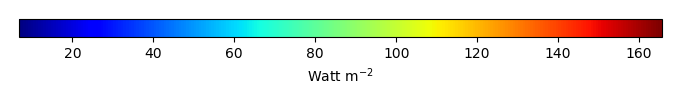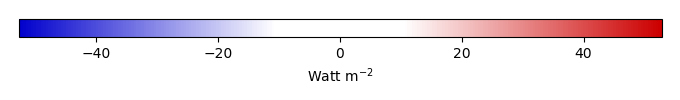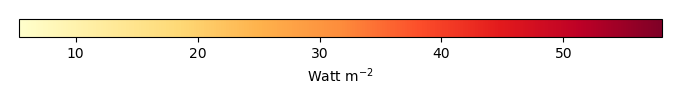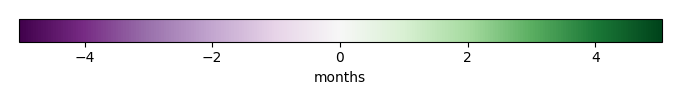# Mean State

Period Mean (original grids) [Watt m-2]
Model Period Mean (intersection) [Watt m-2]
Model Period Mean (complement) [Watt m-2]
Benchmark Period Mean (intersection) [Watt m-2]
Benchmark Period Mean (complement) [Watt m-2]
Bias [Watt m-2]
RMSE [Watt m-2]
Phase Shift [months]
Bias Score 
RMSE Score 
Seasonal Cycle Score 
Spatial Distribution Score 
Interannual Variability Score 
Overall Score 
Benchmark [-] 38.3
CLM5PHSOFF [-] 34.9 34.6 39.6 15.5 -4.88 11.3 1.59 0.326 0.361 0.747 0.979 0.538 0.552
CLM5PHSON [-] 33.9 33.6 39.6 15.5 -5.32 11.4 1.57 0.312 0.367 0.754 0.980 0.532 0.552
Period Mean (original grids) [Watt m-2]
Model Period Mean (intersection) [Watt m-2]
Model Period Mean (complement) [Watt m-2]
Benchmark Period Mean (intersection) [Watt m-2]
Benchmark Period Mean (complement) [Watt m-2]
Bias [Watt m-2]
RMSE [Watt m-2]
Phase Shift [months]
Bias Score 
RMSE Score 
Seasonal Cycle Score 
Spatial Distribution Score 
Interannual Variability Score 
Overall Score 
Benchmark [-] 52.3
CLM5PHSOFF [-] 46.4 46.9 52.8 14.1 -6.00 13.6 1.29 0.428 0.362 0.843 0.990 0.544 0.588
CLM5PHSON [-] 45.3 45.8 52.8 14.1 -7.07 13.8 1.27 0.396 0.379 0.845 0.990 0.530 0.587
Period Mean (original grids) [Watt m-2]
Model Period Mean (intersection) [Watt m-2]
Model Period Mean (complement) [Watt m-2]
Benchmark Period Mean (intersection) [Watt m-2]
Benchmark Period Mean (complement) [Watt m-2]
Bias [Watt m-2]
RMSE [Watt m-2]
Phase Shift [months]
Bias Score 
RMSE Score 
Seasonal Cycle Score 
Spatial Distribution Score 
Interannual Variability Score 
Overall Score 
Benchmark [-] 39.3
CLM5PHSOFF [-] 37.0 37.0 40.4 16.6 -3.46 12.5 1.95 0.406 0.360 0.700 0.928 0.600 0.559
CLM5PHSON [-] 36.6 36.6 40.4 16.6 -3.70 12.2 1.81 0.399 0.383 0.720 0.910 0.579 0.562
Period Mean (original grids) [Watt m-2]
Model Period Mean (intersection) [Watt m-2]
Model Period Mean (complement) [Watt m-2]
Benchmark Period Mean (intersection) [Watt m-2]
Benchmark Period Mean (complement) [Watt m-2]
Bias [Watt m-2]
RMSE [Watt m-2]
Phase Shift [months]
Bias Score 
RMSE Score 
Seasonal Cycle Score 
Spatial Distribution Score 
Interannual Variability Score 
Overall Score 
Benchmark [-] 31.6
CLM5PHSOFF [-] 37.9 38.0 31.5 32.6 6.43 20.6 0.300 0.738 0.559 0.979 0.979 0.768 0.764
CLM5PHSON [-] 34.3 34.2 31.5 32.6 3.60 18.0 0.327 0.799 0.589 0.978 0.972 0.759 0.781
Period Mean (original grids) [Watt m-2]
Model Period Mean (intersection) [Watt m-2]
Model Period Mean (complement) [Watt m-2]
Benchmark Period Mean (intersection) [Watt m-2]
Benchmark Period Mean (complement) [Watt m-2]
Bias [Watt m-2]
RMSE [Watt m-2]
Phase Shift [months]
Bias Score 
RMSE Score 
Seasonal Cycle Score 
Spatial Distribution Score 
Interannual Variability Score 
Overall Score 
Benchmark [-] 23.5
CLM5PHSOFF [-] 29.0 28.9 25.9 15.5 2.93 11.2 1.45 0.373 0.357 0.789 0.275 0.572 0.454
CLM5PHSON [-] 27.8 27.7 25.9 15.5 2.49 11.1 1.43 0.363 0.364 0.791 0.231 0.552 0.444
Period Mean (original grids) [Watt m-2]
Model Period Mean (intersection) [Watt m-2]
Model Period Mean (complement) [Watt m-2]
Benchmark Period Mean (intersection) [Watt m-2]
Benchmark Period Mean (complement) [Watt m-2]
Bias [Watt m-2]
RMSE [Watt m-2]
Phase Shift [months]
Bias Score 
RMSE Score 
Seasonal Cycle Score 
Spatial Distribution Score 
Interannual Variability Score 
Overall Score 
Benchmark [-] 21.4
CLM5PHSOFF [-] 29.8 29.7 21.5 17.6 8.17 18.0 0.585 0.577 0.362 0.927 0.735 0.753 0.619
CLM5PHSON [-] 25.4 25.3 21.5 17.6 3.82 12.6 0.569 0.726 0.468 0.931 0.818 0.743 0.692
Period Mean (original grids) [Watt m-2]
Model Period Mean (intersection) [Watt m-2]
Model Period Mean (complement) [Watt m-2]
Benchmark Period Mean (intersection) [Watt m-2]
Benchmark Period Mean (complement) [Watt m-2]
Bias [Watt m-2]
RMSE [Watt m-2]
Phase Shift [months]
Bias Score 
RMSE Score 
Seasonal Cycle Score 
Spatial Distribution Score 
Interannual Variability Score 
Overall Score 
Benchmark [-] 30.0
CLM5PHSOFF [-] 35.2 35.2 30.5 14.9 4.72 11.9 0.972 0.653 0.460 0.868 0.974 0.680 0.682
CLM5PHSON [-] 35.3 35.3 30.5 14.9 4.16 11.4 0.960 0.671 0.469 0.872 0.973 0.670 0.687
Period Mean (original grids) [Watt m-2]
Model Period Mean (intersection) [Watt m-2]
Model Period Mean (complement) [Watt m-2]
Benchmark Period Mean (intersection) [Watt m-2]
Benchmark Period Mean (complement) [Watt m-2]
Bias [Watt m-2]
RMSE [Watt m-2]
Phase Shift [months]
Bias Score 
RMSE Score 
Seasonal Cycle Score 
Spatial Distribution Score 
Interannual Variability Score 
Overall Score 
Benchmark [-] 29.5
CLM5PHSOFF [-] 33.5 33.5 30.6 14.2 2.74 11.9 1.03 0.588 0.387 0.881 0.979 0.534 0.626
CLM5PHSON [-] 33.5 33.4 30.6 14.2 2.29 11.6 0.992 0.588 0.397 0.884 0.977 0.514 0.626
Period Mean (original grids) [Watt m-2]
Model Period Mean (intersection) [Watt m-2]
Model Period Mean (complement) [Watt m-2]
Benchmark Period Mean (intersection) [Watt m-2]
Benchmark Period Mean (complement) [Watt m-2]
Bias [Watt m-2]
RMSE [Watt m-2]
Phase Shift [months]
Bias Score 
RMSE Score 
Seasonal Cycle Score 
Spatial Distribution Score 
Interannual Variability Score 
Overall Score 
Benchmark [-] 33.0
CLM5PHSOFF [-] 33.6 33.5 32.8 37.1 0.788 17.9 0.272 0.811 0.599 0.982 0.854 0.728 0.762
CLM5PHSON [-] 32.3 32.2 32.8 37.1 -0.0780 17.2 0.288 0.824 0.610 0.981 0.848 0.728 0.767
Period Mean (original grids) [Watt m-2]
Model Period Mean (intersection) [Watt m-2]
Model Period Mean (complement) [Watt m-2]
Benchmark Period Mean (intersection) [Watt m-2]
Benchmark Period Mean (complement) [Watt m-2]
Bias [Watt m-2]
RMSE [Watt m-2]
Phase Shift [months]
Bias Score 
RMSE Score 
Seasonal Cycle Score 
Spatial Distribution Score 
Interannual Variability Score 
Overall Score 
Benchmark [-] 35.1
CLM5PHSOFF [-] 39.3 39.4 36.7 14.1 2.70 10.7 0.551 0.595 0.525 0.952 0.904 0.659 0.693
CLM5PHSON [-] 39.7 39.8 36.7 14.1 2.08 10.6 0.510 0.585 0.527 0.956 0.885 0.646 0.688
Period Mean (original grids) [Watt m-2]
Model Period Mean (intersection) [Watt m-2]
Model Period Mean (complement) [Watt m-2]
Benchmark Period Mean (intersection) [Watt m-2]
Benchmark Period Mean (complement) [Watt m-2]
Bias [Watt m-2]
RMSE [Watt m-2]
Phase Shift [months]
Bias Score 
RMSE Score 
Seasonal Cycle Score 
Spatial Distribution Score 
Interannual Variability Score 
Overall Score 
Benchmark [-] 19.8
CLM5PHSOFF [-] 22.9 22.9 20.7 10.0 2.22 10.2 1.16 0.711 0.526 0.851 0.898 0.690 0.700
CLM5PHSON [-] 22.5 22.5 20.7 10.0 1.77 10.1 1.13 0.714 0.527 0.867 0.870 0.680 0.697
Period Mean (original grids) [Watt m-2]
Model Period Mean (intersection) [Watt m-2]
Model Period Mean (complement) [Watt m-2]
Benchmark Period Mean (intersection) [Watt m-2]
Benchmark Period Mean (complement) [Watt m-2]
Bias [Watt m-2]
RMSE [Watt m-2]
Phase Shift [months]
Bias Score 
RMSE Score 
Seasonal Cycle Score 
Spatial Distribution Score 
Interannual Variability Score 
Overall Score 
Benchmark [-] 23.6
CLM5PHSOFF [-] 45.0 45.0 43.1 14.1 1.98 15.1 0.886 0.605 0.475 0.883 0.866 0.616 0.653
CLM5PHSON [-] 45.0 45.0 43.1 14.1 1.14 14.4 0.858 0.624 0.492 0.887 0.876 0.604 0.663
Period Mean (original grids) [Watt m-2]
Model Period Mean (intersection) [Watt m-2]
Model Period Mean (complement) [Watt m-2]
Benchmark Period Mean (intersection) [Watt m-2]
Benchmark Period Mean (complement) [Watt m-2]
Bias [Watt m-2]
RMSE [Watt m-2]
Phase Shift [months]
Bias Score 
RMSE Score 
Seasonal Cycle Score 
Spatial Distribution Score 
Interannual Variability Score 
Overall Score 
Benchmark [-] 64.3
CLM5PHSOFF [-] 76.7 76.6 66.2 16.5 10.3 18.7 0.559 0.562 0.573 0.946 0.986 0.547 0.698
CLM5PHSON [-] 78.4 78.2 66.2 16.5 10.2 18.6 0.552 0.562 0.573 0.948 0.985 0.543 0.697
Period Mean (original grids) [Watt m-2]
Model Period Mean (intersection) [Watt m-2]
Model Period Mean (complement) [Watt m-2]
Benchmark Period Mean (intersection) [Watt m-2]
Benchmark Period Mean (complement) [Watt m-2]
Bias [Watt m-2]
RMSE [Watt m-2]
Phase Shift [months]
Bias Score 
RMSE Score 
Seasonal Cycle Score 
Spatial Distribution Score 
Interannual Variability Score 
Overall Score 
Benchmark [-] 34.3
CLM5PHSOFF [-] 39.8 39.7 34.2 35.0 5.58 19.3 0.201 0.766 0.592 0.984 0.952 0.775 0.777
CLM5PHSON [-] 37.5 37.5 34.2 35.0 4.47 18.3 0.207 0.791 0.608 0.984 0.976 0.766 0.789
Period Mean (original grids) [Watt m-2]
Model Period Mean (intersection) [Watt m-2]
Model Period Mean (complement) [Watt m-2]
Benchmark Period Mean (intersection) [Watt m-2]
Benchmark Period Mean (complement) [Watt m-2]
Bias [Watt m-2]
RMSE [Watt m-2]
Phase Shift [months]
Bias Score 
RMSE Score 
Seasonal Cycle Score 
Spatial Distribution Score 
Interannual Variability Score 
Overall Score 
Benchmark [-] 24.8
CLM5PHSOFF [-] 34.0 34.0 27.0 14.3 7.01 11.6 0.886 0.504 0.424 0.908 0.869 0.616 0.624
CLM5PHSON [-] 33.8 33.8 27.0 14.3 6.58 11.1 0.872 0.518 0.438 0.909 0.852 0.586 0.624
Period Mean (original grids) [Watt m-2]
Model Period Mean (intersection) [Watt m-2]
Model Period Mean (complement) [Watt m-2]
Benchmark Period Mean (intersection) [Watt m-2]
Benchmark Period Mean (complement) [Watt m-2]
Bias [Watt m-2]
RMSE [Watt m-2]
Phase Shift [months]
Bias Score 
RMSE Score 
Seasonal Cycle Score 
Spatial Distribution Score 
Interannual Variability Score 
Overall Score 
Benchmark [-] 20.7
CLM5PHSOFF [-] 28.3 28.2 21.1 12.1 7.05 10.9 1.03 0.514 0.454 0.856 0.967 0.673 0.653
CLM5PHSON [-] 27.4 27.3 21.1 12.1 6.00 9.62 0.994 0.560 0.479 0.867 0.988 0.652 0.671
Period Mean (original grids) [Watt m-2]
Model Period Mean (intersection) [Watt m-2]
Model Period Mean (complement) [Watt m-2]
Benchmark Period Mean (intersection) [Watt m-2]
Benchmark Period Mean (complement) [Watt m-2]
Bias [Watt m-2]
RMSE [Watt m-2]
Phase Shift [months]
Bias Score 
RMSE Score 
Seasonal Cycle Score 
Spatial Distribution Score 
Interannual Variability Score 
Overall Score 
Benchmark [-] 20.3
CLM5PHSOFF [-] 27.2 27.2 20.6 10.6 6.66 14.7 0.644 0.558 0.433 0.916 0.810 0.695 0.641
CLM5PHSON [-] 23.7 23.7 20.6 10.6 3.05 10.4 0.622 0.685 0.526 0.920 0.905 0.665 0.704
Period Mean (original grids) [Watt m-2]
Model Period Mean (intersection) [Watt m-2]
Model Period Mean (complement) [Watt m-2]
Benchmark Period Mean (intersection) [Watt m-2]
Benchmark Period Mean (complement) [Watt m-2]
Bias [Watt m-2]
RMSE [Watt m-2]
Phase Shift [months]
Bias Score 
RMSE Score 
Seasonal Cycle Score 
Spatial Distribution Score 
Interannual Variability Score 
Overall Score 
Benchmark [-] 30.8
CLM5PHSOFF [-] 35.6 35.6 31.1 10.8 4.38 10.6 0.721 0.642 0.492 0.910 0.994 0.732 0.711
CLM5PHSON [-] 34.2 34.2 31.1 10.8 2.76 9.44 0.757 0.701 0.515 0.903 0.994 0.728 0.726
Period Mean (original grids) [Watt m-2]
Model Period Mean (intersection) [Watt m-2]
Model Period Mean (complement) [Watt m-2]
Benchmark Period Mean (intersection) [Watt m-2]
Benchmark Period Mean (complement) [Watt m-2]
Bias [Watt m-2]
RMSE [Watt m-2]
Phase Shift [months]
Bias Score 
RMSE Score 
Seasonal Cycle Score 
Spatial Distribution Score 
Interannual Variability Score 
Overall Score 
Benchmark [-] 26.8
CLM5PHSOFF [-] 27.7 27.6 27.1 15.2 0.494 8.44 2.24 0.731 0.362 0.641 0.858 0.465 0.570
CLM5PHSON [-] 27.1 27.1 27.1 15.2 0.130 8.33 2.25 0.737 0.366 0.635 0.812 0.454 0.562
Period Mean (original grids) [Watt m-2]
Model Period Mean (intersection) [Watt m-2]
Model Period Mean (complement) [Watt m-2]
Benchmark Period Mean (intersection) [Watt m-2]
Benchmark Period Mean (complement) [Watt m-2]
Bias [Watt m-2]
RMSE [Watt m-2]
Phase Shift [months]
Bias Score 
RMSE Score 
Seasonal Cycle Score 
Spatial Distribution Score 
Interannual Variability Score 
Overall Score 
Benchmark [-] 29.5
CLM5PHSOFF [-] 32.3 32.2 29.9 14.5 2.33 9.38 1.25 0.717 0.433 0.825 0.884 0.550 0.640
CLM5PHSON [-] 31.6 31.6 29.9 14.5 1.66 8.89 1.03 0.743 0.444 0.860 0.807 0.525 0.637
Period Mean (original grids) [Watt m-2]
Model Period Mean (intersection) [Watt m-2]
Model Period Mean (complement) [Watt m-2]
Benchmark Period Mean (intersection) [Watt m-2]
Benchmark Period Mean (complement) [Watt m-2]
Bias [Watt m-2]
RMSE [Watt m-2]
Phase Shift [months]
Bias Score 
RMSE Score 
Seasonal Cycle Score 
Spatial Distribution Score 
Interannual Variability Score 
Overall Score 
Benchmark [-] 28.0
CLM5PHSOFF [-] 32.4 32.3 29.7 12.6 2.46 12.3 0.810 0.561 0.475 0.912 0.931 0.605 0.660
CLM5PHSON [-] 33.1 33.1 29.7 12.6 2.96 12.0 0.745 0.573 0.484 0.918 0.930 0.579 0.661

# Temporally integrated period mean

BENCHMARK MEANMODEL MEANBIASBIAS SCORERMSERMSE SCOREBENCHMARK INTERANNUAL VARIABILITYMODEL INTERANNUAL VARIABILITYINTERANNUAL VARIABILITY SCOREBENCHMARK MAX MONTHMODEL MAX MONTHDIFFERENCE IN MAX MONTHSEASONAL CYCLE SCORESPATIAL TAYLOR DIAGRAMMODEL COLORS# Spatially integrated regional mean

MODEL COLORSREGIONAL MEANANNUAL CYCLEMONTHLY ANOMALYANNUAL CYCLE# All Models

BenchmarkCLM5PHSOFFCLM5PHSON# Data Information

creation_date: Mon Jun 30 23:28:16 PDT 2014

source_file: This product is generated from monthly 1 degree GEWEX SRB Radiation observations